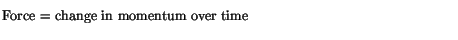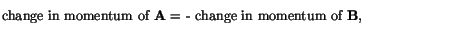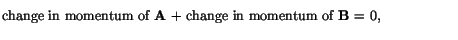Next: Symmetries and Conservation Laws Up: Work and Energy Previous: Mass energy

# Conservation of momentum

As a final point, we mention another fundamental conservation law, which is that of momentum (mass x velocity). Suppose that two objects, A and B, collide. A will exert a force on B, which will change B's momentum according to Newton's 2nd lawSimilarly, B will exert a force on A, which will change A's momentum. These forces, by Newton's 3rd law, are equal and opposite, and therefore the changes in A's and B's momentum are equal and opposite:In other words,We now define the total momentum of the system as the sum of the momentum of A and B. We then haveThis says that the total momentum of a system is conserved. This principle, together with energy conservation, has many far-reaching consequences in many areas of science.

modtech@theory.uwinnipeg.ca
1999-09-29